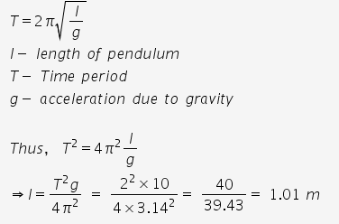# Find the length of seconds pendulum at a place where g = 10 m/s2?

Find the length of seconds pendulum at a place where g = 10 m/s2?

The time required for one oscillation by seconds pendulum is 2 seconds.
T= 2 s